###### eISSN: 2577-8242# Fluid Mechanics Research International JournalTechnical Paper Volume 1 Issue 2

# Aerothermodynamic benefits of mixed exhaust turbofans

#### Syed J Khalid function clickButton(){ var name=document.getElementById('name').value; var descr=document.getElementById('descr').value; var unCopyslNo=document.getElementById('unCopyslNo').value; document.getElementById("mydiv").style.display = "none"; $.ajax({ type:"post", url:"https://medcraveonline.com/captchaCode/server_action", data: { 'name' :name, 'descr' :descr, 'unCopyslNo': unCopyslNo }, cache:false, success: function (html) { //alert('Data Send');$('#msg').html(html); } }); return false; } Verify Captcha Regret for the inconvenience: we are taking measures to prevent fraudulent form submissions by extractors and page crawlers. Please type the correct Captcha word to see email ID.function refreshPage(){ $("#mydiv").load(location.href + " #mydiv"); }$(document).ready(function () { //Disable cut copy paste $('#msg').bind('cut copy paste', function (e) { e.preventDefault(); }); //Disable mouse right click$("#msg").on("contextmenu",function(e){ return false; }); }); .noselect { -webkit-touch-callout: none; /* iOS Safari */ -webkit-user-select: none; /* Safari */ -khtml-user-select: none; /* Konqueror HTML */ -moz-user-select: none; /* Firefox */ -ms-user-select: none; /* Internet Explorer/Edge */ user-select: none; /* Non-prefixed version, currently supported by Chrome and Opera */ cursor: none; }

Gas Turbine Systems Solutions, USA

Correspondence: Syed J Khalid, Gas Turbine Systems Solutions, LLC, Palm Beach Gardens, Florida

Received: September 11, 2017 | Published: November 1, 2017

Citation: Khalid SJ. Aerothermodynamic benefits of mixed exhaust turbofans. Fluid Mech Res Int . 2017;1(2):74-83. DOI: 10.15406/fmrij.2017.01.00009

# Abstract

The preference for mixed exhaust turbofan installations in corporate, regional, and in some commercial airliners is primarily because of the claimed improvement in thrust and Specific Fuel Consumption (SFC) with mixing. This improvement (2 to 3%) has been demonstrated in prior component tests of mixed exhaust configurations in powered wind tunnels. This paper presents back-to-back whole engine cycle studies of both mixed exhaust (Figure 1) and separate exhaust turbofans (Figure 2) with design By-pass Ratios (BPR) of 4, 6, 7, and 8 toward predicting the improvement for the whole engine system. For all configurations modeled the top of climb (TOC,35000’/0.80) net thrust (FN) was kept constant (9000lb) resulting in different core sizes and fan diameters for the clean sheet designs. The model calculations included propulsive efficiency, core efficiency, thermal efficiency, and transmission efficiency. Comparison between mixed exhaust and separate exhaust configurations with the same design BPR showed an altitude SFC advantage with mixed exhaust designs up to a design BPR of 7(2 to 3% for BPR of 4-6), primarily resulting from a higher transmission efficiency. However, for design BPR of 8 the mixed exhaust design showed worse SFC than the separate exhaust turbofan. Also, a comparison of gross thrust (FG) was made at the off-design condition of Sea Level Take-Off (SLTO) when both models were run to the same fuel flow. Again the mixed exhaust design showed a higher gross thrust up to a design BPR of 7, and a loss in thrust for a design BPR of 8. The improvement with mixing (1 to 2% in FG) has been attributed to higher mixed temperature upstream of the common nozzle as explained in the paper. In addition to the back-to-back cycle study, a standalone MATLAB model of the mixer/nozzle was constructed requiring as input the entering separate stream properties available from cycle data. Calculations of the stand alone model showed a similar trend as the cycle model results. The reason for the decrease in performance with mixing for BPR of 8 has been attributed to both reduced mixed gas temperature upstream of the common nozzle and increased mixer pressure loss from increased mixer Mach number. Literature on experimental and numerical investigations of mixer designs toward improving mixer pressure loss while achieving the required mixing effectiveness is listed.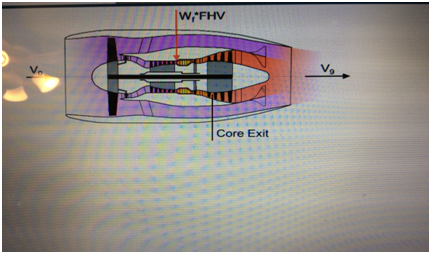Figure 1 Mixed Exhaust Turbofan Schematic.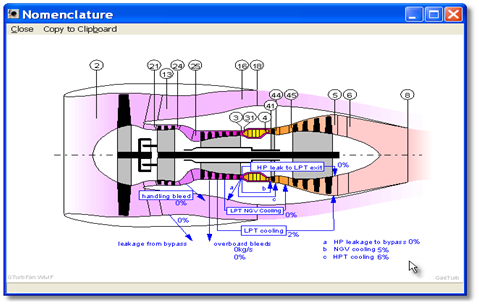Figure 2 Separate Exhaust Turbofan Schematic.

# Nomenclature

$\Delta h$ : Delta enthalpy
A16: Flow area of bypass flow
A6h: flow area on the hot side
A8: Exhaust nozzle throat area
A8: Area at nozzle exit
Abby: Bypass exit area
Acores: Core exit area
ALT: Altitude
Amxd: Mixed Flow Area in the Mixer
as8: Acoustic speed in nozzle throat
asmxd: Mixed gas acoustic speed
Atotal: Total Area aft of the LPT
BPR: Bypass ratio
CPR: High pressure compressor pressure ratio
DeltaPA8: Delta static pressure from ambient at nozzle exit
DpoPmxd: Mixer total pressure loss
ETA_Core: Core efficiency
ETA_Ov: Overall Efficiency
ETA_Prop: Propulsive Efficiency
ETA_therm: Thermal efficiency
ETA_Tran: Transmission Efficiency
Fac1MFF8: Factor 1 in mass flow function equation
Fac2MFF8: Factor 2 in mass flow function equation
FG: Gross Thrust
FgV8: Velocity component of gross thrust for supercritical nozzle
FN: Net Thrust
FPmxd: mixed gas flow parameter
FPRouter: Outer fan pressure ratio
g: Gravitational constant
Hbyp: Total enthalpy of the bypass flow
Hcore: Total enthalpy of the core gas
hmixed: Enthalpy of mixed flow
Hmxd: Total Mixed Enthalpy
HPC: High Pressure Compressor
HPT: High Pressure Turbine
k: Ratio of Specific Heats
kbyp: Specific heat ratio at bypass exit
kcore: Specific heat ratio at core exit
kTmxd: Mixed gas ratio of specific heats corresponding to total temperature
kTsmxd: Mixed gas ratio of specific heats corresponding to static temperature
LPC: Low Pressure Compressor
LPT: Low Pressure Turbine
M0: Flight Mach Number
Mbyp: Bypass exit Mach number
Mcore: Core exit Mach number
MFF8: Nozzle mass flow function
Mmxd: Mixed gas mach number
MOMmxd: Mixed Flow Momentum
N1: Fan Speed
N1C: Corrected fan speed
N2: HPC Speed
N2C: Corrected HPC speed
$\eta core$ : Core efficiency
$\eta ov$ : Overall Efficiency
NPR: Nozzle pressure ratio
$\eta prop$ : Propulsive Efficiency
$\eta therm$ : Thermal Efficiency
$\eta tran$ : Transmission Efficiency
OPR: Overall Compression System Pressure Ratio
P: Total pressure
Pam: Ambient pressure
P16/P6: Bypass stream to core stream total pressure
P24/P21: Booster Pressure Ratio
P3: HPC Exit Total Pressure
Pmxd: Mixed gas total pressure
PRcrit: Critical pressure ratio
Ps16: Bypass Exit Static Pressure
Ps3: HPC Exit Static Pressure
Ps6: LPT Exit Static Pressure
Ps8: Nozzle Throat Static Pressure
Psbyp: Static pressure at the bypass exit
Pscore: Static pressure at core exit
Psmxd: Mixed gas static pressure
Psthroat: Static pressure at nozzle throat
R: Gas constant
RD: Ram Drag
Romxd: Mixed gas density
SFC: Specific Fuel Consumption
SLTO: Sea Level Take-off
T: Total temperature
T3: Compressor Exit Total Temperature
T4: Combustor Exit Total Temperature
TOC: Top of Climb; Altitude, Mach Number
Ts: Static temperature
Ts8: Nozzle throat static temperature
Tsmxd: Mixed gas static pressure
V0: Free stream velocity
V18: Bypass Nozzle Exit Velocity
(V18/V8)ideal: Exhaust Velocity Ratio_Ideal
V8: Core Nozzle Exit Velocity
Vbyp: Velocity at bypass exit
Vcore: Velocity at core exit
Vexit: Exit Velocity
Videal : Ideal exhaust velocity
Vthroat: Velocity at nozzle throat
W2: fan airflow
W25C: Compressor Inlet Corrected Flow
W2C: Engine Inlet Corrected Flow
W3: Compressor Exit Flow
W3C: Compressor Exit Flow Parameter
Wmxd: Mixed Mass Flow in the Mixing Plane
X1: Factor 1 in the exhaust velocity equation
X2: Factor 2 in the exhaust velocity equation

# Introduction

Mixed exhaust turbofans are popular and preferred installations in many corporate and regional aircraft. Also, the single isle airliners, Airbus 319, 320, & 321 series, have demonstrated successful operation with the mixed exhaust turbofan IAE V2500 installation (Figure 3). The preference for this configuration is based on the claim that net thrust and SFC improve with mixed exhaust. However, quantification of these benefits was not adequately available in public domain literature for the whole engine system. In the propulsion and aviation community there is considerable curiosity on knowing the magnitude of these benefits. When design bypass ratio is large, the thermodynamic benefit of mixed exhaust could vanish since the hot flow is a small fraction of the total flow. It is important to establish the criteria which provide guidance on when to select mixed exhaust configurations. Listed references based on powered wind tunnel component tests indicate a thrust improvement of 2 to 3%.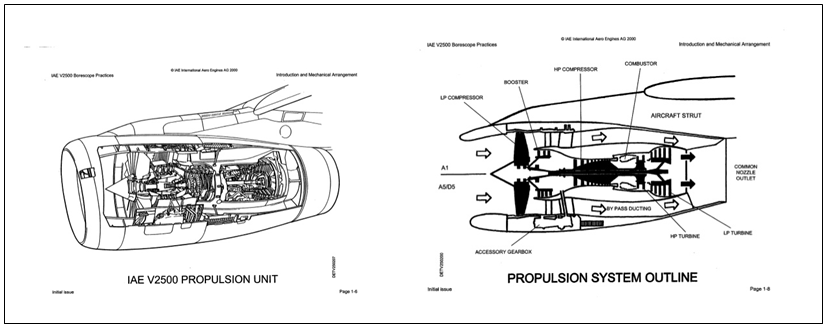Figure 3  V2500 Twin Spool, Mixed Exhaust Turbofan; Courtesy of IAE Corporation.

The purpose of this paper is to analytically investigate the performance improvement possible with mixed exhaust turbofans (Figures 3 & 4) compared to separate exhaust configurations (Figure 5). Figure 4 shows the picture of the GE Honda mixed flow turbofan where the mixer downstream of the LPT mixes the bypass flow and the core flow. A picture of a typical mixer is shown in Figure 6 where the lobes channel the hot flow outward and the cold flow inward for effective mixing. In contrast to the mixed exhaust design, Figure 5 shows the separate exhaust turbofan with separate nozzles for the cold and hot flow. The well proven Gas Turbo 12 performance software1 was selected to model both configurations. A back-to-back comparison of the two types of turbofans was performed when both models were iterated to produce identical design point net thrust, and when both models had identical design point performance parameters including high pressure Compressor Pressure Ratio (CPR), Booster Pressure Ratio (P24/P21), Overall Pressure Ratio (OPR), ByPass Ratio (BPR), Combustor Exit Temperature (T4), cooling flows, and component efficiencies. The comparison was done at top of climb (TOC) max thrust, at cruise conditions, for the altitude SFC “bucket’ of SFC vs FN, and for SLTO gross thrust.Figure 4 GE/Honda Mixed Exhaust Turbofan.Figure 5 PW 4000 Twin Spool, Separate Exhaust Turbofan.

A comparison of gross thrust was made at the off-design condition of sea level, take-off (SLTO) by running both models with the same fuel flow, both incorporating identical component efficiencies. For further confirmation of the comparative results, a standalone MATLAB model of the mixer/nozzle2 was constructed requiring as input the entering separate stream properties obtainable from the cycle data.

# Cycle modeling approach

Separate exhaust configuration

A high level overview of the design point calculation for each engine design is shown in Figure 7. The input parameters which change with each design include the design BPR and the corresponding initial guess for FPRouter. For all design BPR configurations ALT was 35000’, M0 was 0.8, OPR was 40, T4 was 3240R, CPR was 16, and TOC FN was 9000lb, The purpose of the design point calculation is to calculate the flow path areas and determine the scalars for the built- in component maps, so as to achieve the given set of design point performance parameters. The two indicated iterations were added to achieve the given net thrust and the desired ideal velocity ratio, V18/V8)ideal. The W2C iteration was accomplished by varying the map W2C scalar which changed W2C with N1C fixed at 100%. It should be noted that at design point, both the core nozzle and the bypass nozzle operate in the supercritical mode, necessitating the addition of the pressure*area term in the gross thrust calculation2 of Eq. 2. Classically, the preferred V18/V8)ideal for separate exhaust turbofans is 0.80, which is the preferred choice for maximum thrust. Reference 1 gives the rationale based on ideal cycle analysis which indicates that the core nozzle jet velocity has to be higher than bypass nozzle jet velocity. For off-design the design point calculated flow path areas and map scalars were held fixed and performance calculated for the off-design flight conditions and engine power setting.Figure 7 High Level Overview of Separate Exhaust Model Design Point Calculations.

Mixed exhaust configuration

A high level overview of the mixed exhaust design point model is shown in Figure 8. The requirement to have static pressure balance between the cold stream and hot stream (equal static pressures) in the mixing plane is achieved by iterating the area split between the cold stream and the hot stream at the design point. Also, FPRouter is iterated until total pressure ratio, P16/P6 becomes equal to 1. P16/P6 was selected as unity since the study found it to be optimum.3,4 In the mixer, conservation of mass, momentum and energy is performed to obtain the mixed properties and hence the thrust. The mixer calculation should be similar to the one in the isolated MATLAB model2 described later. At the design point the TOC max net thrust was also held constant at 9000 lb by iterating on fan corrected flow as was done in the separate exhaust model of Figure 7. At off-design, the design point calculated flow path areas and map scalars were held fixed and performance calculated for the off-design flight conditions and engine power setting.5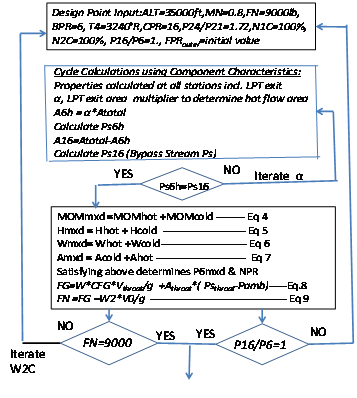Figure 8 High Level Overview of Mixed Exhaust Design Point Calculations showing Iterations for Static Pressure Balance, P16/P6, and TOC Net Thrust.

Some metrics of turbofan performance2,6Model performance calculations included the following parameters which were compared for the mixed exhaust and separate exhaust configurations. The overall efficiency of an aircraft propulsion system is given by:

(1)

where

(2)

(3)

Energy Available: Kinetic Energy of the Jet – Kinetic Energy in Free Stream                        (4)

$\eta prop:\frac{V0\left({V}_{e,i}-V0\right)/g}{\left({V}_{e,i}^{2}-V{0}^{2}\right)/2g}:\frac{2}{1+\frac{{V}_{e,i}}{V0}}$ (5)

(6)

Where dhis is the enthalpy change from core exit conditions to ambient.

$\eta tran:\frac{\eta ov}{\left(\eta core*\eta prop\right)}$ (7)

$V9=\frac{\left(FGcore+FGbyp\right)*g}{W8+W18}$ (8)

(9)

$\eta therm=\frac{\left(\left(0.5*W9*V{9}^{2}-0.5*W0*V{0}^{2}\right)*3600\right)}{\left(2*g*778*WF*FHV\right)}$ (10)

$\text{\hspace{0.17em}}\text{\hspace{0.17em}}\text{\hspace{0.17em}}\text{\hspace{0.17em}}\text{\hspace{0.17em}}\text{\hspace{0.17em}}\text{\hspace{0.17em}}\text{\hspace{0.17em}}\text{\hspace{0.17em}}\text{\hspace{0.17em}}\text{\hspace{0.17em}}\text{\hspace{0.17em}}\text{\hspace{0.17em}}\text{\hspace{0.17em}}\text{\hspace{0.17em}}\text{\hspace{0.17em}}\text{\hspace{0.17em}}\text{\hspace{0.17em}}\text{\hspace{0.17em}}=\eta core*\eta tran$ (11)

(12)

It should be noted that the exhaust velocity for a separate exhaust turbofan is the effective exhaust velocity, V9, given by Eq. 17. As the engine BPR increases, the effective exhaust velocity decreases with the consequent increase in propulsive efficiency (Eq. 14). Also the core size, given by compressor exit flow parameter, W3*sqrt(T3)/P3, decreases. The net result is lower fuel consumption for a given net thrust. Increasing core supercharging with a higher pressure ratio intermediate compressor (booster) pressure ratio also reduces the core size.

# Whole engine performance comparison

Altitude performance analysis

Using the cycle model depicted in Figure 7, design point calculations were first performed for the separate exhaust configuration for design BPR’s of 4, 6, 7 & 8 at 35000’, 0.8 M0, and holding Top of Climb (TOC) Maximum Net Thrust constant at 9000lb. Also, design point calculations were performed for mixed exhaust configurations using the model summarized in Figure 8, for the same bypass ratios and the same TOC Max Net Thrust of 9000lb. Both mixed and separate exhaust configurations had identical design point component efficiencies. For each design BPR, off-design operating lines were generated at 35000’, 0.8 M0. Also, altitude cruise points, assuming a cruise net thrust of 6000 lb, were generated by iterating compressor speed. For the cruise points tabulated in Tables 1 & 2 iterations were also performed to hold fan and booster efficiencies constant at 0.90. This was done to ensure an “apples to apples” comparison between separate and mixed exhaust design since at cruise the migrations in the fan and booster operating points resulted in efficiency variations as much as 2 percent.

SEP EXH

MXD EXH

SEP EXH

MXD EXH

SEP EXH

MXD EXH

TOC_MAX

TOC_MAX

TOC_MAX

TOC_MAX

TOC_MAX

TOC_MAX

Des BPR=4

Des BPR=4

Des BPR=6

Des BPR=6

Des BPR=8

Des BPR=8

ALT

35000

35000

35000

35000

35000

35000

MO

0.8

0.8

0.8

0.8

0.8

0.8

FN

9000

9000

9000

9000

9000

9000

SFC

0.6741

0.6582

0.6221

0.6113

0.5924

0.5978

ETA_Ov

0.2887517

0.295727

0.3128878

0.3184157

0.3285744

0.3256064

ETA_Prop

0.6174

0.6112

0.6748

0.6707

0.7171

0.7187

ETA_Core

0.5552

0.554

0.5536

0.5528

0.5527

0.5522

ETA_Tran

0.8423807

0.8733691

0.837563

0.858812

0.8290192

0.8204439

Table 1  Performance Comparison of Mixed Exhaust Turbofans at TOC and Cruise

SEP EXH

MXD EXH

SEP EXH

MXD EXH

SEP EXH

MXD EXH

CRUISE

CRUISE

CRUISE

CRUISE

CRUISE

CRUISE

Des BPR=4

Des BPR=4

Des BPR=6

Des BPR=6

Des BPR=8

Des BPR=8

ALT

35000

35000

35000

35000

35000

35000

MO

0.8

0.8

0.8

0.8

0.8

0.8

FN

6000

6000

6000

6000

6000

6000

SFC

0.6633

0.6427

0.619559

0.6073

0.5957

0.6071

ETA_Ov

0.2934532

0.302859

0.314171

0.3205129

0.3267542

0.3206185

ETA_Prop

0.6693

0.6671

0.72747

0.7276

0.7685

0.7743

ETA_Core

0.5262

0.5247

0.525561

0.5248

0.5254

0.5259

ETA_Tran

0.8332343

0.8652439

0.8217277

0.8393808

0.8092585

0.7873651

BPROffOes

4.3842

4.6051

6.6852

6.96

9.026

9.2885

Table 2 Performance Comparison of Separate Exhaust Turbofans at TOC and Cruise

Tables 1& 2, Figure 9 show the SFC improvement of 2 to 3% at TOC design point and cruise for design BPR of 4 and 6. Tables 1& 2 include propulsive efficiency, core efficiency, transmission efficiency, and overall efficiency indicating that the improvement with mixed exhaust is from an increase in transmission efficiency. Some references state that mixing induced exhaust velocity uniformity increases propulsion efficiency. However, the cycle data generated for this paper does not indicate an improvement in the calculated propulsive efficiency, but a significant improvement in transmission efficiency(for design BPR of 4 & 6) possibly resulting from the constraints imposed on the LPT exhaust and fan exhaust as cold and hot streams are brought together in the confined space of the mixer. For design BPR of 8, both the design point TOC SFC and cruise SFC for the mixed exhaust design get worse relative to separate exhaust cycle results as graphically presented in Figure 9.Figure 9 Altitude SFC Improvement with Mixed Exhaust.

Comparisons of performance characteristic of SFC vs FN at altitude are presented in Figures 10–12 for design BPR of 6, 7, & 8. The improved SFC with mixed exhaust for design BPR of 6 can be seen in Figure 10. However, as previously stated, for design BPR of 8, mixed exhaust results in degradation in SFC (Figure12). The cycle data for design BPR of 7 shows only slight reduction in SFC with mixed exhaust (Figure 11). The improvement evident from the cycle data corroborates public domain results based on component tests in powered wind tunnels.7‒13Figure 10 Mixed Exhaust Improves Altitude SFC_Des BPR=6.Figure 11 Slight Improvement for design BPR=7.Figure 12 For Design BPR=8 Mixed Exhaust has Worse SFC.

Gross thrust comparison at sea level take-off

At SLTO, to make the comparison meaningful, the mixed exhaust models were run to the same fuel flow as the separate exhaust models. Again the fan and booster efficiencies were held constant at 0.90 for both mixed and separate exhaust configuration for “an apples to apples” comparison. The improvement in SLTO thrust for design BPR of 4 & 6 is shown in Table 3 & Figure 13. The decrease in SLTO thrust for a design BPR of 8 is also shown reflecting the reversal in the benefit as it was also evident at altitude. This reversal is attributed to both the increased mixer Mach number which increases the mixer pressure loss, as well as the reduced mixed temperature. For fixed mixer effectiveness (80% was used in this paper), if BPR is increased, the mixer has to extend to a larger radius, with the attendant increase in mixer wetted area. Also, the decrease in mixed temperature with higher BPR, contributes to higher mixer Mach number. Since pressure loss linearly scales approximately with (Mmixed)^2, higher BPR increases the pressure loss. Mundt C et al.,14 discusses the mixer design with the objective of balancing mixer efficiency and pressure loss primarily by selecting the appropriate number of mixer lobes.

SEP EXH

MXD EXH

SEP EXH

MXD EXH

SEP EXH

MXD EXH

SLTO

SLTO

SLTO

SLTO

SLTO

SLTO

Des BPR=4

Des BPR=4

Des BPR=6

Des BPR=6

Des BPR=8

Des BPR=8

ALT

0

0

0

0

0

0

MO

0

0

0

0

0

0

FN

26334

26850

27972

28324

29685

29204

SFC

0.43485

0.4299

0.3734

0.3688

0.33143

0.3369

ETA_Ov

NA

NA

NA

NA

NA

NA

ETA_Prop

NA

NA

NA

NA

NA

NA

ETA_Core

0.4633

0.464

0.4605

0.4612

0.4588

0.4575

ETA_Tran

NA

NA

NA

NA

NA

NA

BPRoffdes

4.356

4.563

6.73

6.93

9.11

9.1693

Table 3  Gross Thrust Comparison at SLTO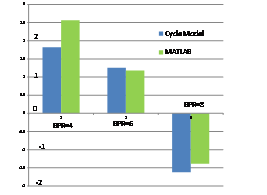Figure 13 SLTO FG Trend Similar to Altitude SFC.

The reason for the benefit with mixed exhaust, as stated in Mundt C et al.,14 is the divergence in constant pressure lines on the h-s diagram. This statement was elaborated and substantiated in this paper by actually performing the calculations for gross thrust for design BPR of 4, 6, & 8 (Figures 14–16). The higher the mixed temperature, the expansion through the nozzle occurs over a greater $\Delta h$ , resulting in a higher exit velocity and thus higher gross thrust. Although the percentage improvement in gross thrust at SLTO is small in comparison to the SFC improvement at altitude, it should be remembered that as the airplane speed increases the percentage net thrust improvement would become higher since ram drag has to be subtracted from gross thrust to get net thrust.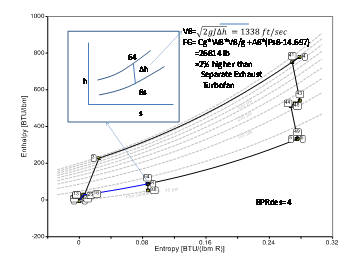Figure 14 Mixed Temperature Benefit_Des BPR=4, SLTO.Figure 15  Mixed Temperatue Benefit_Des BPR=6,SLTO.Figure 16 SLTO Gross Thrust 2% lower with Mixed Exhaust for Design BPR=8.

Mixer/Nozzle stand alone matlab model calculations

To confirm the cycle model results at sea-level take-off conditions, a standalone MATLAB model of the mixer/nozzle (Figure 17) was constructed requiring as input the entering cold stream and hot stream properties which were obtainable from the cycle model data. This cycle model input had different specific heats and k for the cold and hot streams (function of temperature). The calculations are delineated below showing the solution of the conservation equations of mass, momentum, and energy to determine mixed properties. The algorithm was also used in Khalid S2 the mixer/nozzle calculations of a mixed exhaust turbofan illustrative example. The converging nozzle calculations depended upon whether the the nozzle pressure ratio, NPR, was above critical or below critical. In the event NPR>critical, gross thrust calculation included the pressure times area term in addition to the velocity term. The velocity term included the gross thrust coefficient, CFG. The value of CFG used was identical to that used in GasTurb12 for consistency.3 Mixer pressure loss for different BPR configurations was obtained by scaling the base pressure loss for a design BPR of 6 with the factor (Mmixed/Mmixed, BPR:6)^2.Figure 17 Schematic of the Isolated Mixer/Nozzle.

Results from the stand alone MATLAB program are compared to the SLTO cycle model results in Figure 13 showing reasonable agreement especially considering the small differences in gross thrust. Reference 1 also reports improvement from mixing from a standalone model where the conservation equations were solved. However, it used constant specific heats and a k of 1.4 for both cold and hot stream. Although this simplified model of Reference 1 predicted improvement from mixing, it did not predict decreasing benefit with increasing BPR. The analysis in this paper predicts a performance loss from mixing at BPR of 8 or greater. All modern high BPR turbofans have separate exhausts.15‒18

Mixing Plane Calculation: It is required to satisfy conservation laws noting that

Continuity: ${\stackrel{˙}{m}}_{core}+{\stackrel{˙}{m}}_{byp}={\stackrel{˙}{m}}_{noz}$ (13)

Energy: ${h}_{mixed}=\frac{\left({H}_{core}+{H}_{byp}\right)}{{\stackrel{˙}{m}}_{noz}}$ (14)

Momentum: $\left(P{s}_{byp}×{A}_{byp}+{\stackrel{˙}{m}}_{byp}{V}_{byp}\right)+\left(P{s}_{core}{A}_{core}+{\stackrel{˙}{m}}_{core}{V}_{core}\right)$ (15)

$T{t}_{mixed}=\frac{\left({H}_{core}+{H}_{byp}\right)}{C{p}_{core}{\stackrel{˙}{m}}_{core}+C{p}_{byp}{\stackrel{˙}{m}}_{byp}}$ (16)

Bypass + Core Stream Momentum can also be expressed as:

$P{s}_{byp}{A}_{byp}\left(1+{k}_{byp}{M}_{byp}{}^{2}\right)+P{s}_{core}{A}_{core}\left(1+{k}_{core}{M}_{core}{}^{2}\right)$ (17)

Mixed Stream Momentum after using Equation of State and continuity becomes:

${\stackrel{˙}{m}}_{mixed}×R×\frac{T{s}_{mixed}}{{V}_{mixed}}+{\stackrel{˙}{m}}_{mixed}\frac{{V}_{mixed}}{g}$ (18)

Substituting for Tsmixed

$Ts=Tt-\frac{{V}^{2}}{2gJCp}$ (19)

Also by substituting Eq. 28 in Eq 27 and equating to Eq. 26 we obtain:

$\begin{array}{l}{\stackrel{˙}{m}}_{mixed}×R×\frac{T{t}_{mixed}}{{V}_{mixed}}-{\stackrel{˙}{m}}_{mixed}{V}_{mixed}\frac{R}{2gJC{p}_{mixed}}+{\stackrel{˙}{m}}_{mixed}\frac{{V}_{mixed}}{g}\\ =\left(P{s}_{byp}{A}_{byp}\left(1+{k}_{byp}{M}_{byp}^{2}\right)\right)+\left(P{s}_{core}{A}_{core}\left(1+{k}_{core}{M}_{core}^{2}\right)\right)\end{array}$ (20)

Multiplying Eq. 29 by Vmxdand rearranging we get the quadratic equation:

$\begin{array}{l}{V}_{mxd}^{2}\left[{\stackrel{˙}{m}}_{mxd}/g-{R}_{mxd}*{\stackrel{˙}{m}}_{mxd}/\left(2gJC{p}_{mxd}\right)\right]\\ -{V}_{mxd}\left[{A}_{core}P{s}_{core}\left(1+{k}_{core}{M}_{core}^{2}\right)+\\ +{A}_{byp}P{s}_{byp}\left(1+{k}_{byp}{M}_{byp}^{2}\right)\right]+{\stackrel{˙}{m}}_{mxd}*{R}_{mxd}*{T}_{mxd}=0\end{array}$ (21)

From the above equation in the form ax^2 +bx+c , Vmxd:(-b-sqrt(b^2-4ac))/2a    (31)

Tsmxd:Tmxd-Vmxd^2/(2*32.174*778*cpmxd)
kTsmxd:1.5476E-08*Tsmxd^2 - 9.8690E-05*Tsmxd + 1.4396E+00
asmxd:sqrt(32.174*kTsmxd*53.3*Tsmxd)
Mmxd:Vmxd/asmxd
Romxd:Wmxd/(Amxd*Vmxd)
Psmxd:Romxd*53.3*Tsmxd
kTmxd:1.5476E-08*Ttmxd^2 - 9.8690E-05*Ttmxd + 1.4396E+00
Pmxd:Psmxd*(1+((kTmxd-1)/2)*Mmxd^2)^(kTmxd/(kTmxd-1))
FPmxd:Wmxd*sqrt(Ttmxd)/Pmxd
DpoPmxd:.015*(Mmxd/0.3031)^2

Nozzle Calculation:

# Summary and conclusion

The analytical predictions in this paper, using both whole engine system model and stand alone mixer/nozzle model, indicate a modest performance improvement for mixed exhaust configurations of moderate bypass ratio. The 2 to 3% in SFC improvement corroborates published results based on component tests conducted in powered wind tunnels. The reason for the SFC improvement at altitude, evident from cycle data, is primarily higher transmission efficiency with mixed exhaust. The higher gross thrust at SLTO with mixing is the result of higher mixed exhaust temperature.

Whole engine test data showing a back-to-back comparison of mixed exhaust and separate exhaust designs is not available since it is impractical (possibly impossible) to conduct a meaningful test keeping the performance parameters of both configurations almost identical. The best comparative predictions for the whole engine system, therefore, have to be determined analytically using physics based models.

This paper used both a well proven performance software with typical component maps as well as a specially constructed stand alone physics based model both showing similar results. This adds to the confidence in the predicted results. Literature survey was also included citing the conclusions of other investigators.1,7,8,12 The results of this analytical study either corroborated the previously published results based on component tests and/or provided additional insight. It should be noted that the Energy Efficient Engine (E3) reports of both GE and Pratt et al.,8,19 included a mixer for the bypass and core streams. However, the design BPR in both reports was less than 7 possibly for the same reason concluded in this paper that the pay-off from mixing occurs when the design BPR is 7 or lower. The modern high BPR engines all have separate exhausts.20,21

# Acknowledgments

The author wishes to express his thanks to the following:

1. Joaquim Kurzke and Daniel Weitraub of GasTurb Gmbh for navigational instructions on GasTurb12
2. Pratt & Whitney, Unit of United Technologies Corporation for permission to use their copyright material
3. GE Aviation for permission to use their copyright information.

# Conflicts of interest

Author declares that there is no conflict of interest.

# References©2017 Khalid. This is an open access article distributed under the terms of the, which permits unrestricted use, distribution, and build upon your work non-commercially.